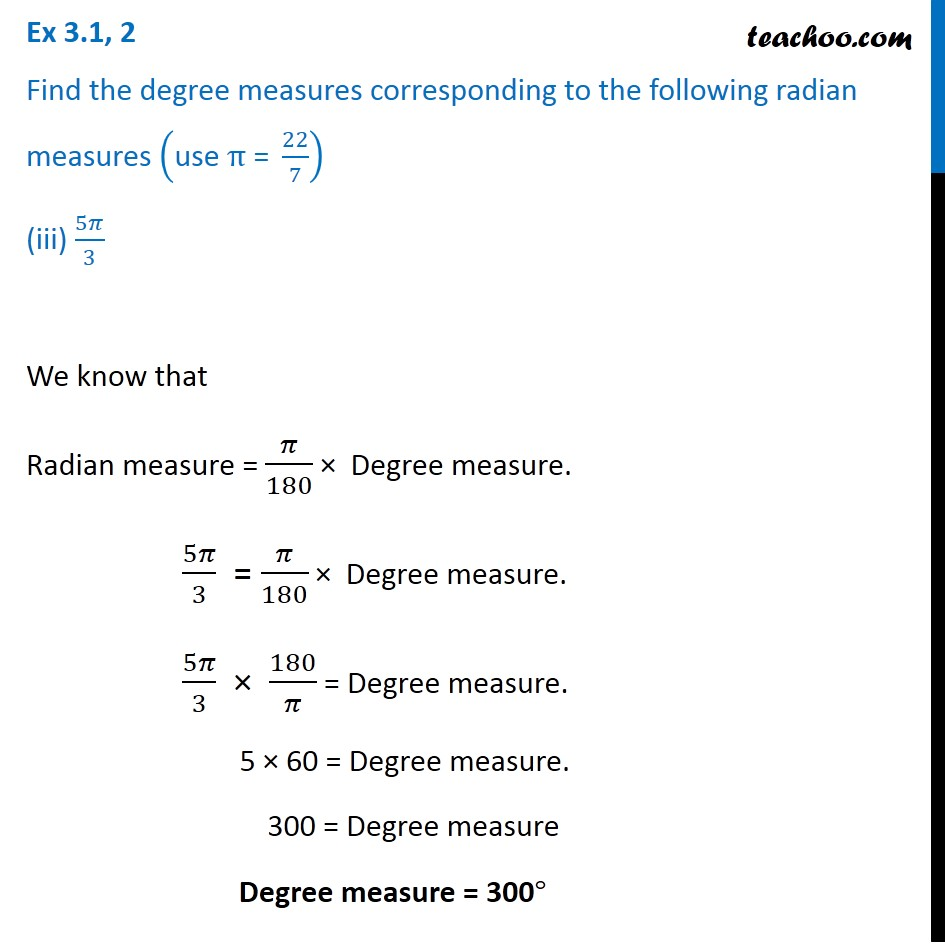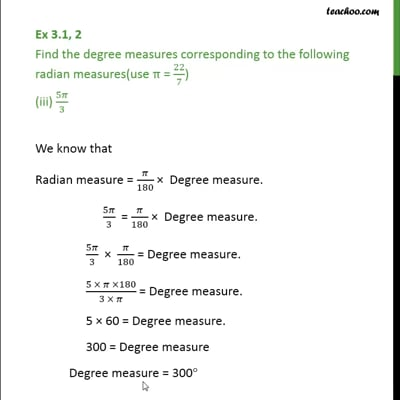Ex 3.1

Chapter 3 Class 11 Trigonometric Functions
Serial order wiseThis video is only available for Teachoo black users

Introducing your new favourite teacher - Teachoo Black, at only ₹83 per month

### Transcript

Ex 3.1, 2 Find the degree measures corresponding to the following radian measures ("use " π" = " 22/7) (iii) 5𝜋/3 We know that Radian measure = 𝜋/180 × Degree measure. 5𝜋/3 = 𝜋/180 × Degree measure. 5𝜋/3 × 180/𝜋 = Degree measure. 5 × 60 = Degree measure. 300 = Degree measure Degree measure = 300°